# CBSE Class 12 Physics Chapter 7 Revision Notes

Chapter 7: Alternating Current Revision Notes

• AC stands for alternating current.
• It’s a current that alternates in magnitude and direction, and does so on a regular basis.

I = I0 sin ωt or I = I0 cosωt where, I0 = AC’s peak or maximum value.

• Effective Value (rms Value of AC): It is defined as the AC value over a complete cycle that generates the same amount of heat in a given resistor as steady current in the same resistor at the same time during a complete cycle.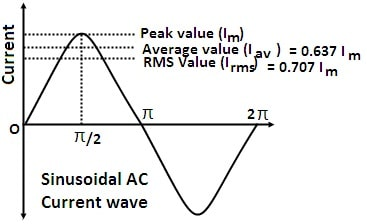Source

• The effective or rms value of AC is 70.7 percent of the peak value of current.
• AC’s Average or Mean Value: It is defined as the AC value that would send the same amount of charge through a circuit in half-cycle as steady current would send in the same amount of time.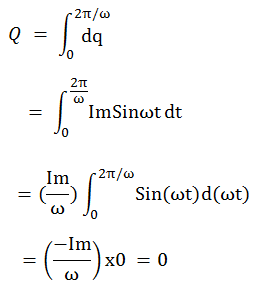Source

• The average or mean value of AC is 63.7 percent of the peak value.
• The mean value of AC in a complete cycle will be zero.
• Voltage or alternating emf It is the emf that alternately and periodically changes in magnitude and direction.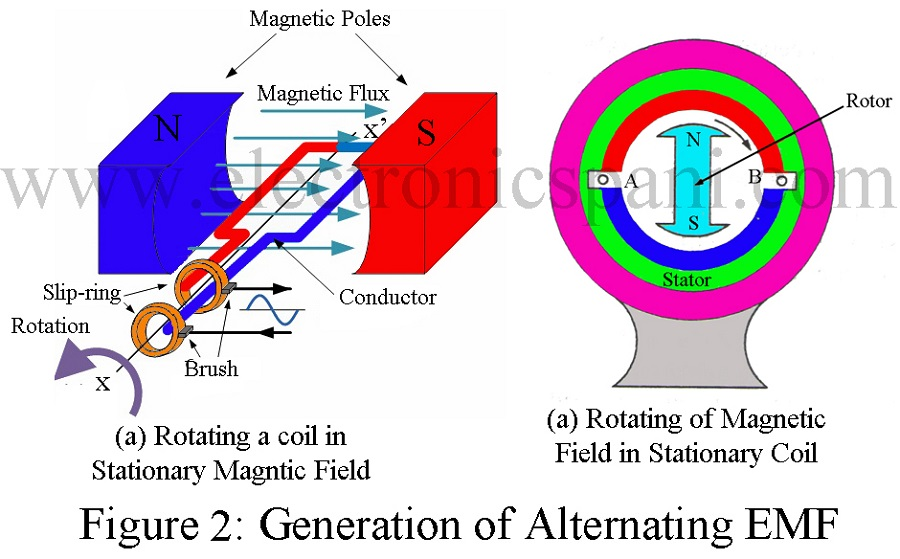Source

Diagrams are used to represent both AC voltage and AC current, as shown below: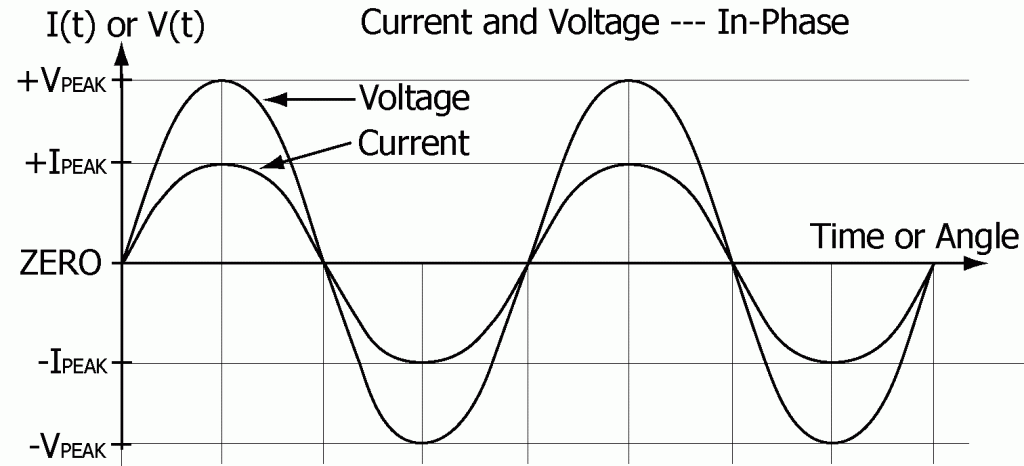Source

• Inductive Reactance (n.d.) is a term that refers to (XL) Inductive reactance is the property of an inductor that opposes current flow.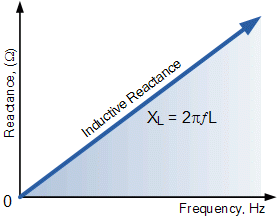Source

• Capacitive Reactance (Xc) is the property of a capacitor that opposes the flow of alternating current.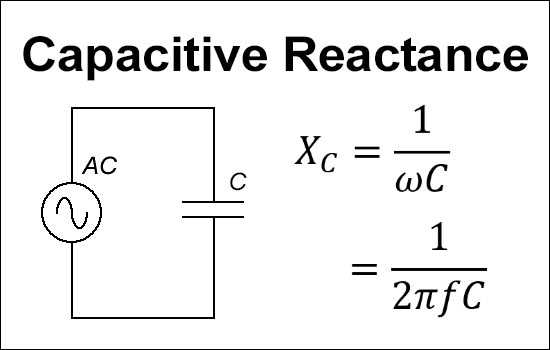Source

• Wattless Current, also known as idle current, is the current in an AC circuit when the average power consumption is zero.
]]>